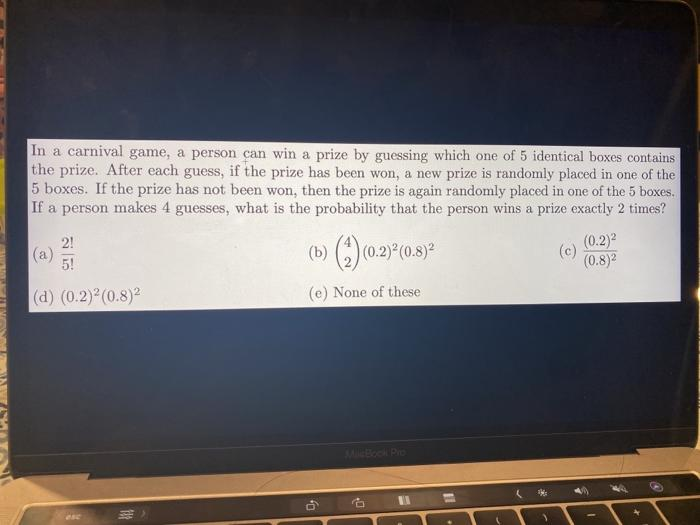# Question In a carnival game, a person can win a prize by guessing which one of 5 identical boxes contains the prize. After each guess, if the prize has been won, a new prize is randomly placed in one of the 5 boxes. If the prize has not been won, then the prize is again randomly placed in one of the 5 boxes. If a person makes 4 guesses, what is the probability that the person wins a prize exactly 2 times? 2! (a) (0.2) (0.2) 5! (c) (0.8)2 (a) (0.2) (0.8) (e) None of these * 11QVHEKH The Asker · Probability and StatisticsTranscribed Image Text: In a carnival game, a person can win a prize by guessing which one of 5 identical boxes contains the prize. After each guess, if the prize has been won, a new prize is randomly placed in one of the 5 boxes. If the prize has not been won, then the prize is again randomly placed in one of the 5 boxes. If a person makes 4 guesses, what is the probability that the person wins a prize exactly 2 times? 2! (a) (0.2) (0.2) 5! (c) (0.8)2 (a) (0.2) (0.8) (e) None of these * 11
More
Transcribed Image Text: In a carnival game, a person can win a prize by guessing which one of 5 identical boxes contains the prize. After each guess, if the prize has been won, a new prize is randomly placed in one of the 5 boxes. If the prize has not been won, then the prize is again randomly placed in one of the 5 boxes. If a person makes 4 guesses, what is the probability that the person wins a prize exactly 2 times? 2! (a) (0.2) (0.2) 5! (c) (0.8)2 (a) (0.2) (0.8) (e) None of these * 11